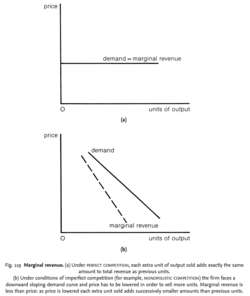# marginal revenue

Also found in: Acronyms, Encyclopedia, Wikipedia.

## Marginal revenue

The change in total revenue as a result of producing one additional unit of output.

## Marginal Revenue

The revenue that a company generates over what it previously generated for each additional unit of output. For example, suppose a company generates \$1000 in revenue from 100 units of a product (in other words \$10 per unit). In order to sell 101 units it may have to reduce its price to \$9.99 per unit. In this case, its revenue becomes \$1008.99. Thus, the marginal revenue is \$8.99.

## marginal revenue

The extra revenue generated by selling one additional unit of a good or service. For example, if a firm can sell 10 units of a product at a price of \$25 per unit, total revenue is \$250. If, in order to sell 11 units, it must reduce the price to \$24, total revenue rises to 11 × \$24, or \$264. Thus, the marginal revenue of the 11th unit is \$264 - \$250, or \$14.

## marginal revenue

the extra revenue that is obtained by a firm from the sale of additional units of product. If firms are profit maximizers they will seek to equate marginal revenue with MARGINAL COST to establish that price output/sales combination which yields an optimal return. See BUSINESS OBJECTIVE.
Collins Dictionary of Business, 3rd ed. © 2002, 2005 C Pass, B Lowes, A Pendleton, L Chadwick, D O’Reilly and M AffersonFig. 119 Marginal revenue. (a) Under PERFECT COMPETITION, each extra unit of output sold adds exactly the same amount to total revenue as previous units.

Under conditions of imperfect competition (for example, MONOPOLISTIC COMPETITION) the firm faces a downward sloping demand curve and price has to be lowered in order to sell more units. Marginal revenue is less than price: as price is lowered each extra unit sold adds successively smaller amounts than previous units.

## marginal revenue

the addition to TOTAL REVENUE from the sale of one extra unit of output. Under conditions of PERFECT COMPETITION, the firm faces a horizontal DEMAND CURVE at the going market price (marginal revenue = price). See Fig. 119 (a) . Marginal revenue interacts with MARGINAL COST in determining the level of output at which the firm achieves its objective of PROFIT MAXIMIZATION. See AVERAGE REVENUE, ELASTICITY OF DEMAND, KINKED DEMAND CURVE, MONOPOLY.
Collins Dictionary of Economics, 4th ed. © C. Pass, B. Lowes, L. Davies 2005
References in periodicals archive ?
In a pedagogically useful reconstruction of the theory of factor pricing, he first contrasts the timeless neoclassical general equilibrium theory, in which prices of factors of production correspond to the factor's marginal revenue product and are determined simultaneously with final output prices, with the Austrian analysis of price determination in the evenly rotating economy (ERE).
The marginal revenue of a minister or advisor becomes negative if we add overheads like air-conditioning, transport, ministerial payroll, and so on.
Second, Mises did not use marginal revenue in his analysis of margin monopoly.
If marginal revenue is greater than marginal cost, then this implies that the very next unit the producer makes will bring in more revenue than it costs to make.
Marketing analysis indicates that total revenue for AX-12 at 10,000 units will be \$200,000, and total revenue at 12,000 units will be \$235,000; thus, Beta faces declining marginal revenue. It will incur a \$5 per-unit variable cost in the production of AX-12, but no incremental fixed cost.
production up to the point where the marginal revenue of making one more
The objective can be accomplished through estimating the production function for dates and deriving marginal product and marginal revenue functions of water resources, estimating the value of the marginal revenue of water resources in the domestic market price and export price (social price for dates), and measuring the impact of changes in the prices of water resources on the water quantities used in the production of dates.
In this case, marginal revenue depends explicitly on the number of workers employed, which changes the incentives for a firm in a noncooperative bargaining setting.
(5) The well-known Shapley value, in this case the expected marginal revenue of each song, averaging over all of its possible arrival orders in the bundle, provides a theoretical solution to the revenue-sharing problem.
The first part is the marginal revenue for the subscribers, and the second part concerns the congestion effects which is [partial derivative][Q.sub.i]/[partial derivative][d.sub.i] < 0 if [d.sub.i] > [d.sub.i.sup.*] or 0 otherwise.

Site: Follow: Share:
Open / Close